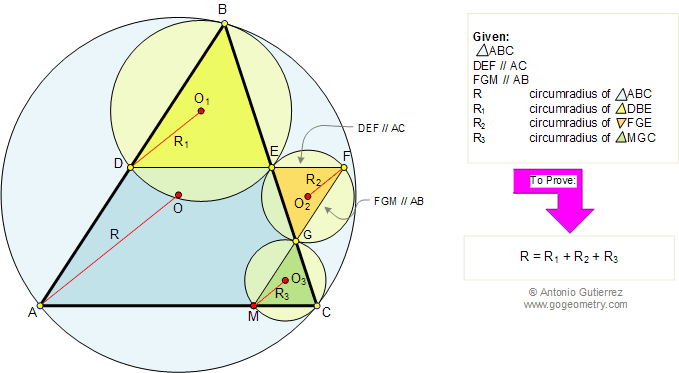# Problem 94. Similar Triangles, Circumradii, Parallel Lines. High School, College

 In the figure below, given a triangle ABC, line DEF parallel to AC and line FGM parallel to AB. If R, R1, R2, and R3, are the circumradii of triangles ABC, DBE, FGE, and MGC respectively, prove that R = R1 + R2 + R3. View or post a solution .FACTS AND HINTS:

Geometry problem solving is one of the most challenging skills for students to learn. When a problem requires auxiliary construction, the difficulty of the problem increases drastically, perhaps because deciding which construction to make is an ill-structured problem. By “construction,” we mean adding geometric figures (points, lines, planes) to a problem figure that wasn’t mentioned as "given."

1. SIMILAR TRIANGLES:
Proposition:
Corresponding angles of similar triangles are congruent.

Home | Geometry | Search | Problems | Similarity | Circumcircle | Parallelograms | All Problems | 91-100 | Visual Index | Email | View or post a solution | by Antonio Gutierrez